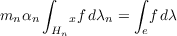#### Vol. 20, No. 1, 1967

 Download this articleFor screen For printingRecent Issues Vol. 325: 1 Vol. 324: 1  2 Vol. 323: 1  2 Vol. 322: 1  2 Vol. 321: 1  2 Vol. 320: 1  2 Vol. 319: 1  2 Vol. 318: 1  2Online Archive Volume: Issue:The Journal Subscriptions Editorial Board Officers Contacts Submission Guidelines Submission Form Policies for Authors ISSN: 1945-5844 (e-only) ISSN: 0030-8730 (print) Special Issues Author Index To Appear Other MSP Journals
Jessen’s theorem on Riemann sums for locally compact groups

### Kenneth Allen Ross and Karl Robert Stromberg

Vol. 20 (1967), No. 1, 135–147
##### Abstract

Throughout this paper G denotes a locally compact group and {Hn} denotes an increasing sequence of closed subgroups of G whose union H is dense in G. For each n,Δn denotes the modular function on Hn and Δ denotes the modular function on G. Then limnΔn(x) = Δ(x) for each x H. For each n,λn denotes a left Haar measure on Hn and λ denotes a left Haar measure on G. For a function f on G and an x in G,xf denotes the function xf(y) = f(xy). The main theorem states that if Δn is the restriction of Δ to Hn for all sufficiently large n, then there is a “normalizing” sequence {αn} of positive numbers such that for every f in L1(G,λ)(1)

for λ-locally almost all x in G. The hypotheses regarding the Δn’s and Δ hold in all cases known to the authors. In particular, they hold if the Hn’s are unimodular (hence if they are Abelian, compact, or discrete) or if the Hn’s are open subgroups or normal subgroups. If G is the compact group0,1with addition modulo 1, if the Hn’s are the finite groups {k2n : 0 k 2n 1} with counting measure λn, and if αn = 2n, then the left side of (1) is a Riemann sum and (1) becomes Jessen’s theorem.

Primary: 22.20
##### Milestones